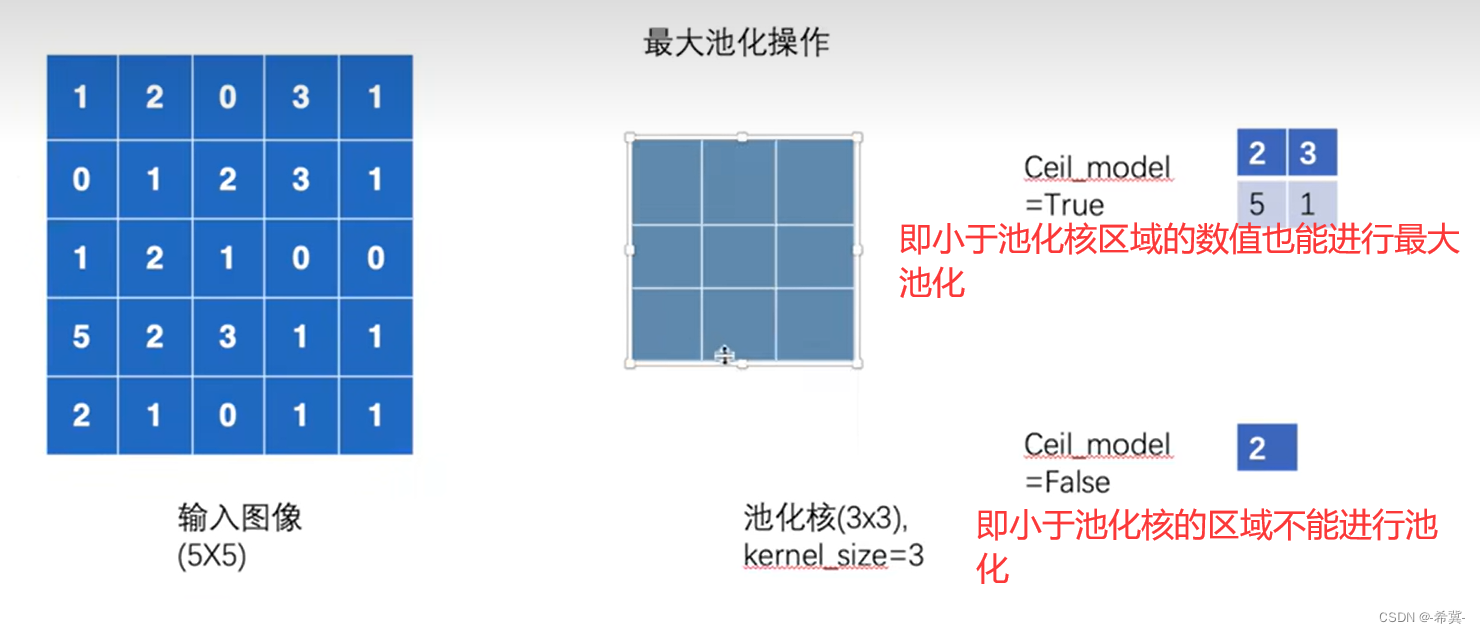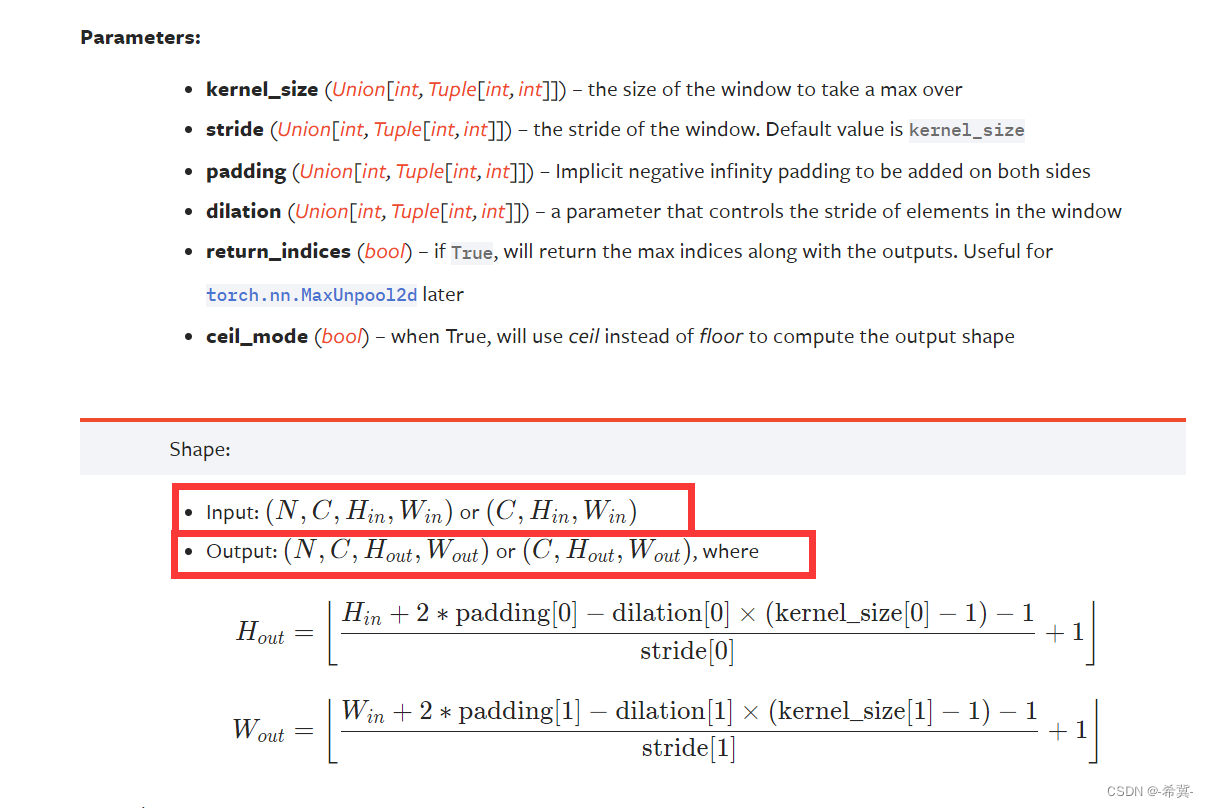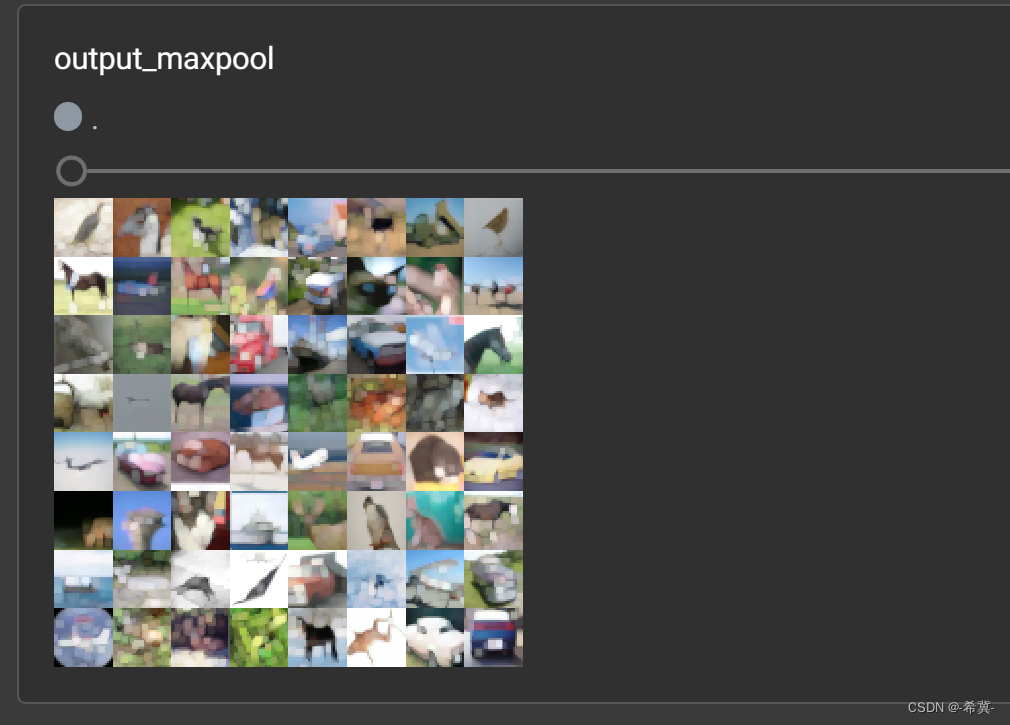## Pytorch深度学习-----神经网络之池化层用法详解及其最大池化的使用

chatgpt/2023/9/26 14:02:58

# 系列文章目录

PyTorch深度学习——Anaconda和PyTorch安装
Pytorch深度学习-----数据模块Dataset类
Pytorch深度学习------TensorBoard的使用
Pytorch深度学习------Torchvision中Transforms的使用（ToTensor，Normalize，Resize ，Compose，RandomCrop）
Pytorch深度学习------torchvision中dataset数据集的使用（CIFAR10）
Pytorch深度学习-----神经网络的基本骨架-nn.Module的使用
Pytorch深度学习-----神经网络的卷积操作
Pytorch深度学习-----神经网络之卷积层用法详解

### 文章目录

• 系列文章目录
• 一、池化操作是什么？
• 二、torch.nn.MaxPool2d介绍
• 1.相关参数
• 2.最大池化处理上述矩阵并验算结果
• 3.最大池化处理CIFAR10数据集图片

# 一、池化操作是什么？

`特征降维`：通过减小特征图的尺寸，减少了后续层的计算量和参数数量，有助于降低过拟合风险。
`提取主要特征`：通过选择最大值或求平均值，池化操作可以提取出最显著的特征，有助于保留重要信息并抑制噪声。# 二、torch.nn.MaxPool2d介绍

## 1.相关参数

torch.nn.MaxPool2d(kernel_size, stride=None, padding=0, dilation=1, return_indices=False, ceil_mode=False)

kernel_size：表示池化核的大小，类型为int 或者元组。
stride=None：表示步长的大小，与卷积层不同，池化层步长大小`默认为kernel_size的大小`
dilation=1：表示设置核的膨胀率，默认 dilation=1，即如果kernel_size =3，那么核的大小就是3×3。如果dilation = 2，kernel_size =3×3，那么每列数据与每列数据，每行数据与每行数据中间都再加一行或列数据，数据都用0填充，那么核的大小就变成5×5。
return_indices=False：表示用来控制要不要返回最大值的索引位置，如果为true，那么要记住最大池化后最大值的所在索引位置，后面上采样可能要用上，为false则不用记住位置。
ceil_mode=False：表示计算输出结果形状的时候，是使用向上取整还是向下取整。即要不要舍弃无法覆盖核的大小的数值。
`注意` 输入和输出的input需要为NCHW或者CHW## 2.最大池化处理上述矩阵并验算结果

``````import torch
from torch import nninput = torch.tensor([[1,2,0,3,1],[0,1,2,3,1],[1,2,1,0,0],[5,2,3,1,1],[2,1,0,1,1]],dtype=float)  # 使用dtype将此矩阵的数字变为浮点型
# 准备的参数情况
print(input.shape)  # torch.Size([5, 5])
# 进行reshape
input = torch.reshape(input,(1,5,5))  # 修改shape为chw
print(input.shape)  # torch.Size([1, 5, 5])# 搭建神经网络并进行池化操作
class Lgl(nn.Module):def __init__(self):super(Lgl,self).__init__()self.maxpool2 = nn.MaxPool2d(kernel_size=3,ceil_mode=True)def forward(self,input):return self.maxpool2(input)# 实例化
l = Lgl()
output = l(input)
print(output)
``````
``````torch.Size([5, 5])
torch.Size([1, 5, 5])
tensor([[[2., 3.],[5., 1.]]], dtype=torch.float64)
``````

2,3,5,1 刚好符合ceil_mode=True时的情况

``````import torch
from torch import nninput = torch.tensor([[1,2,0,3,1],[0,1,2,3,1],[1,2,1,0,0],[5,2,3,1,1],[2,1,0,1,1]],dtype=float)  # 使用dtype将此矩阵的数字变为浮点型
# 准备的参数情况
print(input.shape)  # torch.Size([5, 5])
# 进行reshape
input = torch.reshape(input,(1,5,5))  # 修改shape为chw
print(input.shape)  # torch.Size([1, 5, 5])# 搭建神经网络并进行池化操作
class Lgl(nn.Module):def __init__(self):super(Lgl,self).__init__()self.maxpool2 = nn.MaxPool2d(kernel_size=3,ceil_mode=False)def forward(self,input):return self.maxpool2(input)# 实例化
l = Lgl()
output = l(input)
print(output)``````
``````torch.Size([5, 5])
torch.Size([1, 5, 5])
tensor([[[2.]]], dtype=torch.float64)
``````

## 3.最大池化处理CIFAR10数据集图片

``````在这里插入代码片
``````### 师从美国四院院士|遗传学老师赴哥伦比亚大学访问交流

H老师为省公派访学&#xff0c;目标为美国知名高校&#xff0c;最终我们获得了哥伦比亚大学的邀请函&#xff0c;导师是美国科学院院士、美国艺术与科学院院士、美国微生物学院院士、美国科学促进会会士等四个学会的院士&#xff0c;堪称学术界的超级大牛。 H老师背景&#xff…